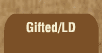Mathematics and Dyslexia

Tips for Learning the Times Tables

Tools for the Times Tables & Workbook

Home > Math > times tables practice > Nines In Order (all of them)

## The Nines Times Tables -all of them in order

The digits of the numbers that you can divide by nine always add up to nine. For 18, 1 + 8 is 9; for 27, 2 + 7 is 9, etc. etc.

If you're thinking, "I did this with the small and big ones already," that's right. Can you put them all together? It should take less than two minutes of your life if you know them automatically, so rather than come up with reasons not to, spend that time getting them typed in.

This page should be as fast and easy as the 5s or the 1s. If you're having to stop and think a lot here, it won't get easier when these are mixed up with other numbers!

1. 9 x 0=
2. 1 x 9=
3. 9 x 2=
4. 3 x 9=
5. 9 x 4=
6. 5 x 9=
7. 9 x 6=
8. 7 x 9=
9. 9 x 8=
10. 9 x 9=
11. 10 x 9=
12. 9 x 9=
13. 9 x 7=
14. 6 x 9=
15. 9 x 8=